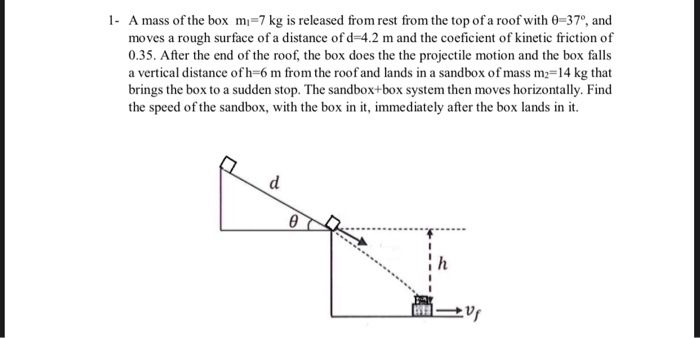# Question Solved1 Answer1- A mass of the box mi=7 kg is released from rest from the top of a roof with O=370, and moves a rough surface of a distance of d=4.2 m and the coeficient of kinetic friction of 0.35. After the end of the roof, the box does the the projectile motion and the box falls a vertical distance of h=6 m from the roof and lands in a sandbox of mass m2=14 kg that brings the box to a sudden stop. The sandbox+box system then moves horizontally. Find the speed of the sandbox, with the box in it, immediately after the box lands in it. Boty USTranscribed Image Text: 1- A mass of the box mi=7 kg is released from rest from the top of a roof with O=370, and moves a rough surface of a distance of d=4.2 m and the coeficient of kinetic friction of 0.35. After the end of the roof, the box does the the projectile motion and the box falls a vertical distance of h=6 m from the roof and lands in a sandbox of mass m2=14 kg that brings the box to a sudden stop. The sandbox+box system then moves horizontally. Find the speed of the sandbox, with the box in it, immediately after the box lands in it. Boty US
More
Transcribed Image Text: 1- A mass of the box mi=7 kg is released from rest from the top of a roof with O=370, and moves a rough surface of a distance of d=4.2 m and the coeficient of kinetic friction of 0.35. After the end of the roof, the box does the the projectile motion and the box falls a vertical distance of h=6 m from the roof and lands in a sandbox of mass m2=14 kg that brings the box to a sudden stop. The sandbox+box system then moves horizontally. Find the speed of the sandbox, with the box in it, immediately after the box lands in it. Boty US